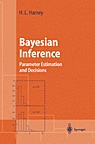# Prof. Hanns Ludwig Harney

## book

### Bayesian Inference

H.L. Harney, November 3, 2005

The book provides a generalization of Gaussian error intervals to situations where the data follow non-Gaussian distributions. This occurs whenever the interesting parameter is just above background. It ususally occurs in multiparametric data where the histogram of the events contains empty bins. Then the error intervals of the extracted parameters cannot be defined via Gaussian statistics. Furthermore, the chi-squared-criterion cannot be used to decide on the validity of the model. It has been generalized. --- The book is based on Bayes' theorem, symmetry considerations and differential geometry. In addition to solutions of practical problems, the text provides an epistemic insight: The logic of quantum mechanics is the logic of unbiased inference from counting events. However, no knowledge of quantum mechanics is required. The text, examples and exercises are written at an introdutory level.Bayesian Inference
Parameter Estimation and Decisions